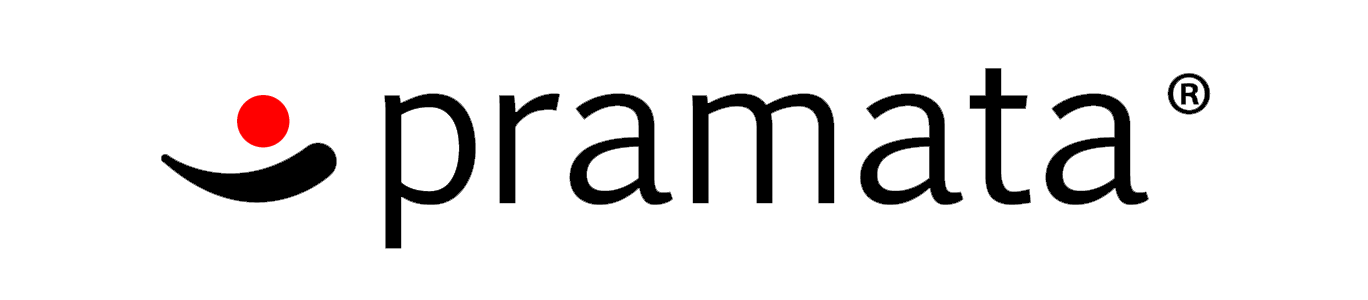Xsquare And Two Arrays
Tag(s):

## Basic Programming, Dynamic Programming, Easy, Implementation

Problem
Editorial
Analytics

Xsquare loves to play with arrays a lot. Today, he has two arrays named as A and B. Each array consists of N positive integers.

Xsquare has decided to fulfill following types of queries over his array A and array B.

• 1 L R : Print the value of AL + BL+1 + AL+2 + BL+3 + ... upto Rth term.
• 2 L R : Print the value of BL + AL+1 + BL+2 + AL+3 + ... upto Rth term.

### Input

First line of input contains two space separated integers N and Q denoting the size of arrays ( A , B ) and number of queries respectively. Next N lines of input contains N space separated integers denoting array A. Next line of input contains N space separated integers denoting array B. Next Q lines of input contains Q queries (one per line). Each query has one of the above mentioned types.

### Output

Output consists of Q lines, each containing answer to the corresponding query.

### Constraints

• 1 ≤ N,Q ≤ 105
• 1 ≤ Ai,Bi ≤ 109
• 1 ≤ L,R ≤ N
SAMPLE INPUT
5 5
1 2 3 4 5
5 4 3 2 1
1 1 5
2 1 5
1 2 4
2 2 4
1 3 5


SAMPLE OUTPUT
15
15
9
9
10

Explanation

Q1 : A + B + A + B + A = 1 + 4 + 3 + 2 + 5 = 15 Q2 : B + A + B + A + B = 5 + 2 + 3 + 4 + 1 = 15 Q3 : A + B + A = 2 + 3 + 4 = 9 Q4 : B + A + B = 4 + 3 + 2 = 9 Q5 : A + B + A = 3 + 2 + 5 = 10

Time Limit: 5.0 sec(s) for each input file.
Memory Limit: 256 MB
Source Limit: 1024 KB
Marking Scheme: Marks are awarded when all the testcases pass.
Allowed Languages: Bash, C, C++, C++14, Clojure, C#, D, Erlang, F#, Go, Groovy, Haskell, Java, Java 8, JavaScript(Rhino), JavaScript(Node.js), Julia, Kotlin, Lisp, Lisp (SBCL), Lua, Objective-C, OCaml, Octave, Pascal, Perl, PHP, Python, Python 3, R(RScript), Racket, Ruby, Rust, Scala, Swift, Swift-4.1, TypeScript, Visual Basic

## CODE EDITOR

Initializing Code Editor...

## This Problem was Asked inChallenge Name

Pramata Hiring Challenge

OTHER PROBLEMS OF THIS CHALLENGE
• Basic Programming > Bit Manipulation